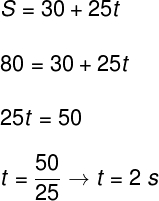Mechanics

# uniform motion

Uniform motion (MU) occurs in a straight line and with constant velocity, therefore, it has no acceleration.

Uniform motion is displacement that occurs in a straight line and with constant speed , thus covering equal distances in equal time intervals. In uniform motion, there is no acceleration .

Suppose a vehicle moves in uniform motion with a speed of 30 km/h. In a time interval of 30 minutes (0.5 hour), this vehicle travels 15 km; in 60 minutes (1 hour), it travels 30 km; in 2 hours, therefore, 60 km.

All uniform motion is rectilinear. If the body is moving in a circular path with constant velocity, there will always be an acceleration pointing to the center of the curves ( centripetal acceleration ), so this type of motion cannot be categorized as uniform, but uniform circular.

## Uniform motion formulas

In uniform motion, the position varies with time constantly. We can calculate the position a mobile will be in after a time interval (t). To do so, we must know its initial position (S 0 ) and the speed (v) with which this body moves. Once you have identified this information, simply use it in the following formula: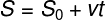S – final position of the mobile
0 – initial position of the mobile
v – speed of the mobile
t – time interval

To use this formula, it is necessary to remember the units used for each of the above quantities according to the International System of Units (SI). According to the SI positions are given in meters (m), velocity is given in meters per second (m/s) and the time interval is measured in seconds (s).

Furthermore, the notion of a frame of reference is fundamental for the correct application of the above formula. In physics, a frame of reference is the position in which the observer is. It is from this position, denoted as 0, that we define the initial position of a mobile. Look at the following figure:

The frame of reference indicates the direction of movements.

In the figure above, we have a referential (0) adopted and three pieces of furniture that move in different directions. For the silver vehicle that is to the left of the frame, we have a negative initial position (S 0 = -12 m) – the same is true for the red vehicle (S 0 = -8 m). For the second silver vehicle , we have a positive starting position (S 0 = 15 m). Note that the choice of reference directly affects the initial positions of the furniture, in addition to determining the direction of its movement. Look at the following figure:

In the figure above, we can see that the choice of reference (0) caused the first silver car, located on the left, to be approaching, while the other vehicles are moving away. These are the two possible classifications of uniform movements : when a piece of furniture approaches the referential, we call its movement regressive ; if the mobile moves away, the movement is progressive.

## Average speed

The average speed of a movement is calculated by the ratio between the displacement traveled and the time interval. This is an important quantity for the study of uniform motion.

The formula used to calculate the average velocity is simply a different way of writing the uniform motion position time function shown earlier. Check out how the formula for average speed is defined: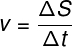v – average velocity
ΔS – displacement
Δt – time interval

In the above formula, ΔS is called displacement and it measures the distance between the final (S f ) and initial positions of a mobile (S 0 ), therefore, ΔS = S f – 0 . The time interval between the passage of the mobile through the initial and final positions is given by Δt , calculated by the expression Δt = t f – t 0 .

speed units

As we know, the SI unit of speed is the meter per second (m/s), however, it is common to come across situations where the reported speed is in another unit, such as the kilometer per hour (km/h). Conversion between these two units is quite simple. Check out the image below:

To convert from meters per second to kilometers per hour, we multiply the value by the factor 3.6. To perform the inverse transformation, simply divide the velocity by the same factor.

## Uniform motion graphics

Uniform motion can be represented in the form of graphs of position versus time with ascending or descending lines Ascending lines correspond to progressive movements , that is, movements in which the furniture moves away from its reference. Descending lines are used for regressive movements , those in which the furniture approaches its references.

Observe the graph below, which represents the movement of a mobile that moves with constant velocity, moving away from its reference.

The graph below represents a mobile moving in regressive motion. Watch:

Another important and very useful information concerns the calculation of the displacement traveled by the mobile from the graphs. However, this method only works for graphs of velocity versus time (v(t)), such as the following:

In the graph shown above, for example, the painted area is equivalent to the area of ​​a rectangle, which can be calculated by the following formula: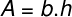A – area
b – base edge
h – height

## Uniform motion exercises

1) An observer notices that a vehicle, initially at a distance of 30 m, is moving away with a constant speed of 90 km/h. In relation to this movement, he responds:

a) What is the motion classification of this vehicle? Explain.

b) Determine the time function of this vehicle’s position in SI units.

c) At what instant of time will this vehicle be at position 80 m?

Resolution:

a) As the vehicle moves away from its observer, its movement is progressive. In addition, as its speed is constant, it is a uniform and progressive movement.

b) To determine the time function of the movement, we need to read the exercise and identify some quantities, such as initial position and velocity. By doing so, it is possible to see that the trajectory of the vehicle was started at position 30 m , therefore, 0 = 30 m. Its speed, 90 km/h, needs to be expressed in SI. To do this, just divide it by the factor 3.6, so v = 25 m/s .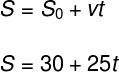c) We can calculate the instant when the vehicle will pass the position S = 80 m by substituting this number in the formula found above. Look: